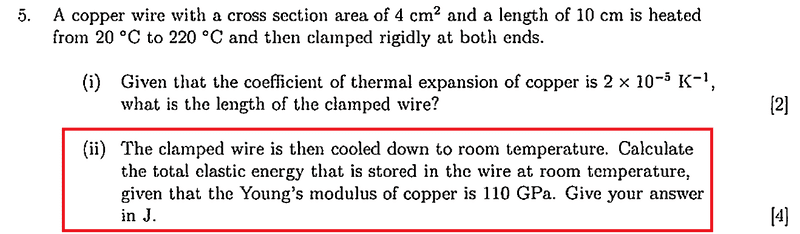## Homework Statement## The Attempt at a Solution

I tried to use the equation introduced in the class, E=σε/2
ε=αΔT =2*10-5*200 = 4*10-3
and by σ=Yε, I get σ=110*109*4*10-3 = 4.4*109
so E=(4*10-3) * (4.4*109 ) /2 = 880000J
Why am I wrong?
The correct answer is 35.2 J

#### Attachments

•